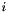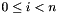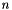MPQC  2.3.1
The KeyVal Library

The KeyVal class provides a means for users to associate keywords with values. ParsedKeyVal is a specialization of KeyVal that permits keyword/value associations in text such as an input file or a command line string.

The package is flexible enough to allow complex structures and arrays as well as objects to be read from an input file.

# Assignment

As an example of the use of ParsedKeyVal, consider the following input:

x_coordinate = 1.0
y_coordinate = 2.0
x_coordinate = 3.0


Two assignements will be made. The keyword x_coordinate will be associated with the value 1.0 and the keyword y_coordinate will be assigned to 2.0. The third line in the above input will have no effect since x_coordinate was assigned previously.

# Keyword Grouping

Lets imagine that we have a program which needs to read in the characteristics of animals. There are lots of animals so it might be nice to catagorize them by their family. Here is a sample format for such an input file:

reptile: (
alligator: (
legs = 4
extinct = no
)
python: (
legs = 0
extinct = no
)
)
bird: (
owl: (
flys = yes
extinct = no
)
)


This sample illustrates the use of keyword = value assignments and the keyword grouping operators ( and ). The keywords in this example are

reptile:alligator:legs
reptile:alligator:extinct
reptile:alligator:legs
reptile:python:size
reptile:python:extinct
bird:owl:flys
bird:owl:extinct


The :'s occuring in these keywords break the keywords into smaller logical units called keyword segments. The sole purpose of this is to allow persons writing input files to group the input into easy to read sections. In the above example there are two main sections, the reptile section and the bird section. The reptile section takes the form reptile : ( keyword = value assignments ). Each of the keywords found between the parentheses has the reptile: prefix attached to it. Within each of these sections further keyword groupings can be used, as many and as deeply nested as the user wants.

Keyword grouping is also useful when you need many different programs to read from the same input file. Each program can be assigned its own unique section.

# Array Construction

Input for an array is specified in the input by forming a keyword group. The name of the group is the name of the array and the grouped keywords are the integers, such that, whereis the number of elements in the array. For example, an array, called array, of length 3 could be given as follows:

array: (
0 = 5.4
1 = 8.9
2 = 3.7
)


The numbers 0, 1, and 2 in this example are keyword segments which serve as indices of array. However, this syntax is somewhat awkward and array construction operators have been provided to simplify the input for this case. The following input is equivalent to the above input:

array = [ 5.4 8.9 3.7 ]


More complex arrays than this can be imagined. Suppose an array of complex numbers is needed. For example the input

carray: (
0: ( r = 1.0  i = 0.0 )
1: ( r = 0.0  i = 1.0 )
)


could be written as

carray: [
(r = 1.0 i = 0.0)
(r = 0.0 i = 1.0)
]


which looks a bit nicer than the example without array construction operators.

Furthermore, the array construction operators can be nested in about every imaginable way. This allows multidimensional arrays of complicated data to be represented. Here is an example of input for a lower triangular array:

ltriarray = [ [ 5.4  ]
[ 0.0 2.8 ]
[ 0.1 0.0 3.7 ] ]


# Table Construction

Although the array construction operators will suit most requirements for enumerated lists of data, in some cases the input can still look ugly. This can, in some cases, be fixed with the table construction operators, { and }.

Suppose a few long vectors of the same length are needed and the data in the ith element of each array is related or somehow belong together. If the arrays are so long that the width of a page is exceeded, then data that should be seen next to each other are no longer adjacent. The way this problem can be fixed is to arrange the data vertically side by side rather than horizontally. The table construction operators allows the user to achieve this in a very simple manner.

balls: (
color    = [  red      blue     red   ]
diameter = [   12       14       11   ]
material = [  rubber  vinyl   plastic ]
bounces  = [  yes      no       no    ]
coordinate = [[ 0.0  0.0  0.0]
[ 1.0  2.0 -1.0]
[ 1.0 -1.0  1.0]]
)


can be written

balls: (
{ color diameter material bounces     coordinate} =
{  red     12    rubber    yes     [ 0.0  0.0  0.0]
blue    14    vinyl     no      [ 1.0  2.0 -1.0]
red     11    plastic   no      [ 1.0 -1.0  1.0] }
)


The length and width of the table can be anything the user desires.

Value Substitution

Occasionally, a user may need to repeat some value several times in an input file. If the value must be changed, it would be nice to only change the value in one place. The value substitution feature of ParsedKeyVal allows the user to do this. Any place a value can occur the user can place a $. Following this a keyword must be given. This keyword must have been assigned before the attempt is made to use its value in a value substitution. Here is an example illustrating most of the variable substition features: default:linewidth = 130 testsub: ( ke: ( ke_1 = 1 ke_2 = 2 ke_3: ( ke_31 = 31 ke_32 = 32 ) ) kx =$ke
r1 = 3.0
r2 = $r1 linewidth =$:default:linewidth
)


is the same as specifying

testsub: (
ke: (
ke_1 = 1
ke_3: (
ke_31 = 31
ke_32 = 32
)
ke_2 = 2
)
linewidth = 130
r2 = 3.0
r1 = 3.0
kx: (
ke_1 = 1
ke_2 = 2
ke_3: (
ke_31 = 31
ke_32 = 32
)
)
)


It can be seen from this that value substitution can result in entire keyword segment hierarchies being copied, as well as simple substitutions.

# Expression Evaluation

Suppose your program requires several parameters x1, x2, and x3. Furthermore, suppose that their ratios remain fixed for all the runs of the program that you desire. It would be best to specify some scale factor in the input that would be the only thing that has to be changed from run to run. If you don't want to or cannot modify the program, then this can be done directly with ParsedKeyVal as follows

scale = 1.234
x1 = ( $:scale * 1.2 ) x2 = ($:scale *  9.2 )
x3 = ( $:scale * -2.0 )  So we see that to the right of the <tt>=</tt>'' the characters ('' and ‘)’' are the expression construction operators. This is in contrast to their function when they are to the left of the ‘=’', where they are the keyword grouping operators. The expression must be binary and the data is all converted to double. If you use the expression construction operators to produce data that the program expects to be integer, you will certainly get the wrong answers (unless the desired value happens to be zero). # Objects An instance of an object can be specified by surrounding it's classname with the <tt><</tt>'' and>'' operators immediately after the keyword naming the data. A pointer to a single object can be associated with multiple keywords by using value substitution. This is accomplished by holding references to all objects once they are read in. Consider a linked list class, A, which reads from the keyword next a reference to an object of class A. Input for such an object, read from keyword a1, follows: a1<A>: ( next<A>: ( next<B>: ( bdata = 4 next<A>:() ) ) ) a2 =$:a1


The a1 list would contain two A objects followed by a B object followed by another A object. The a2 list refers to exactly the same object as a1 (not a copy of a1).

Generated at Sun Jan 26 2020 23:33:05 for MPQC 2.3.1 using the documentation package Doxygen 1.8.16.